Скачать презентацию Householder Transformations Example DEF is called Householder matrix

8a1523e00f0b71fdc6d5a63f7c145ae9.ppt

• Количество слайдов: 19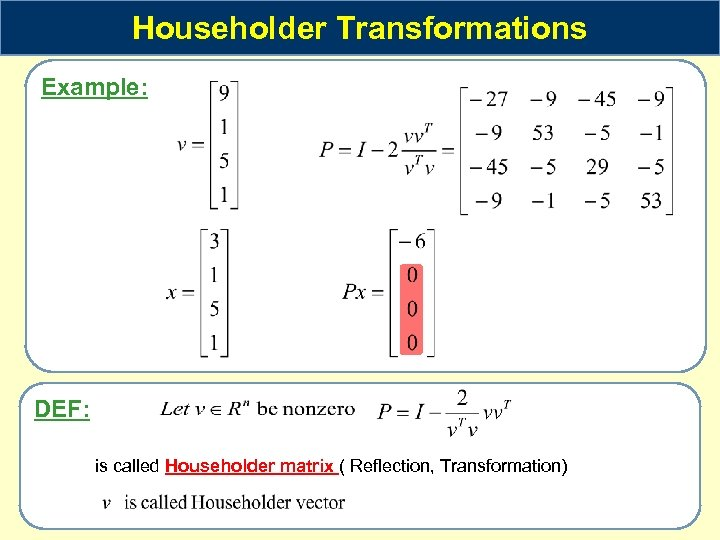Householder Transformations Example: DEF: is called Householder matrix ( Reflection, Transformation)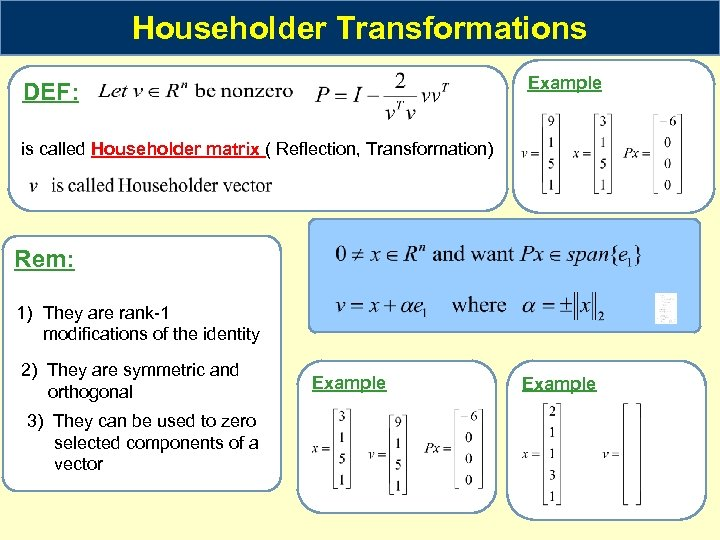Householder Transformations Example DEF: is called Householder matrix ( Reflection, Transformation) Rem: 1) They are rank-1 modifications of the identity 2) They are symmetric and orthogonal 3) They can be used to zero selected components of a vector Example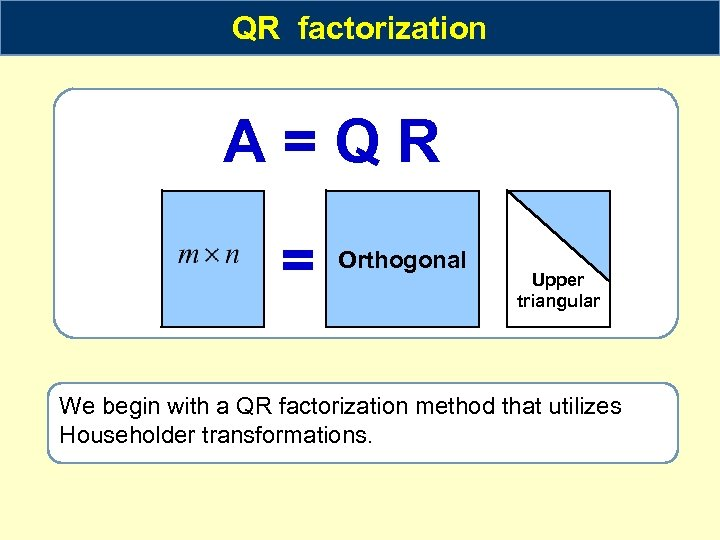QR factorization A=QR = Orthogonal Upper triangular We begin with a QR factorization method that utilizes Householder transformations.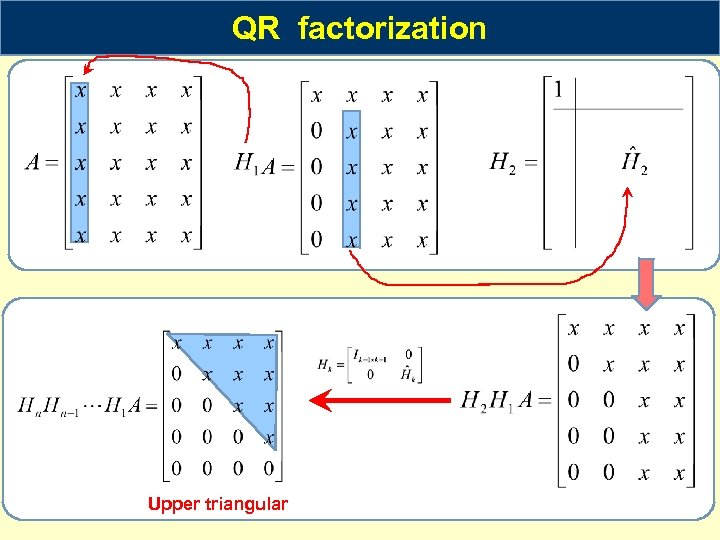QR factorization Upper triangular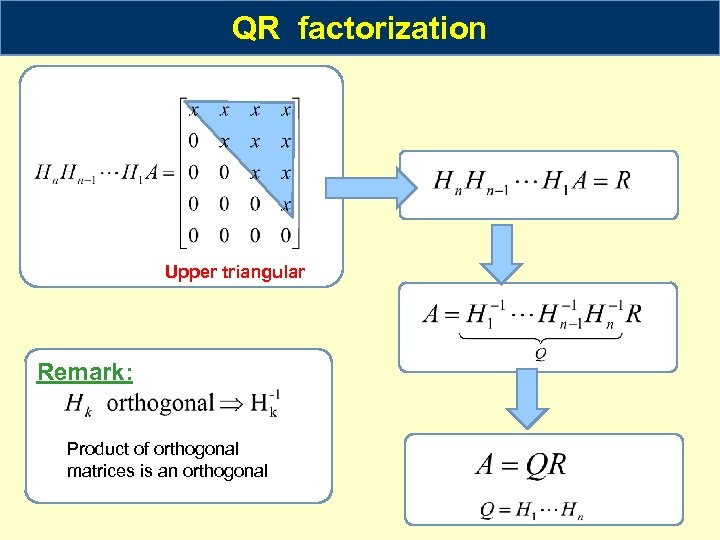QR factorization Upper triangular Remark: Product of orthogonal matrices is an orthogonal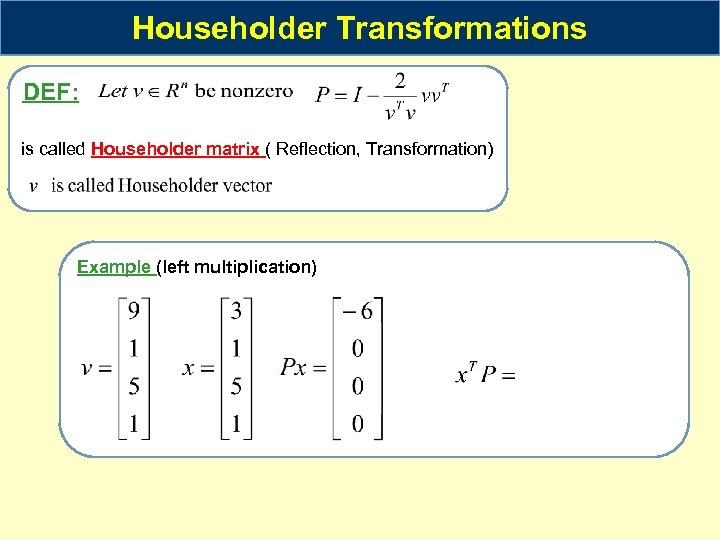Householder Transformations DEF: is called Householder matrix ( Reflection, Transformation) Example (left multiplication)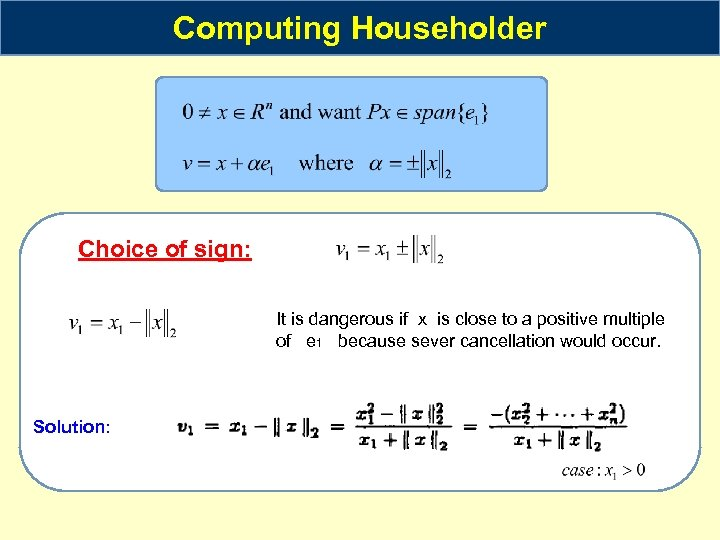Computing Householder Choice of sign: It is dangerous if x is close to a positive multiple of e 1 because sever cancellation would occur. Solution: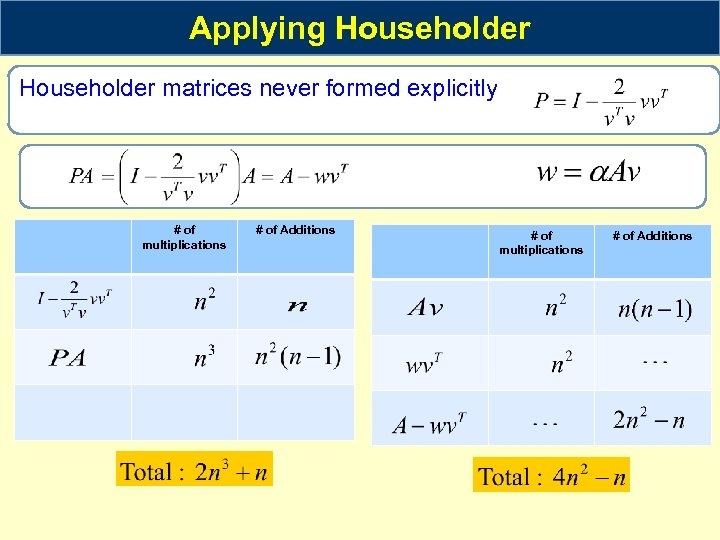Applying Householder matrices never formed explicitly # of multiplications # of Additions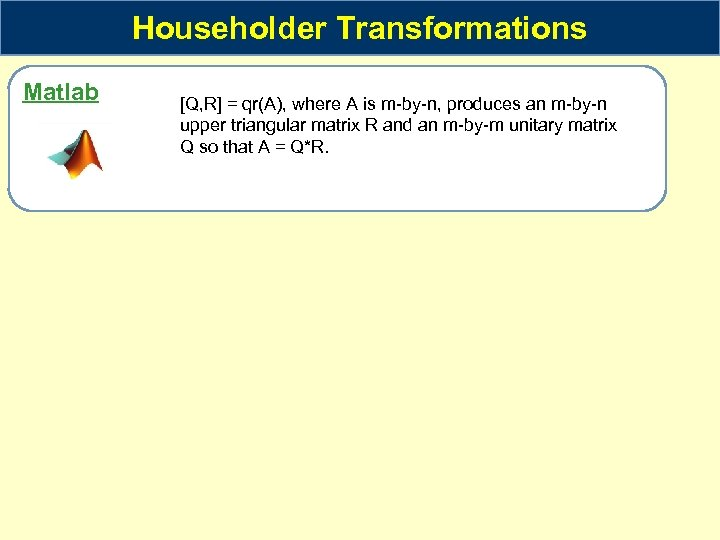Householder Transformations Matlab [Q, R] = qr(A), where A is m-by-n, produces an m-by-n upper triangular matrix R and an m-by-m unitary matrix Q so that A = Q*R.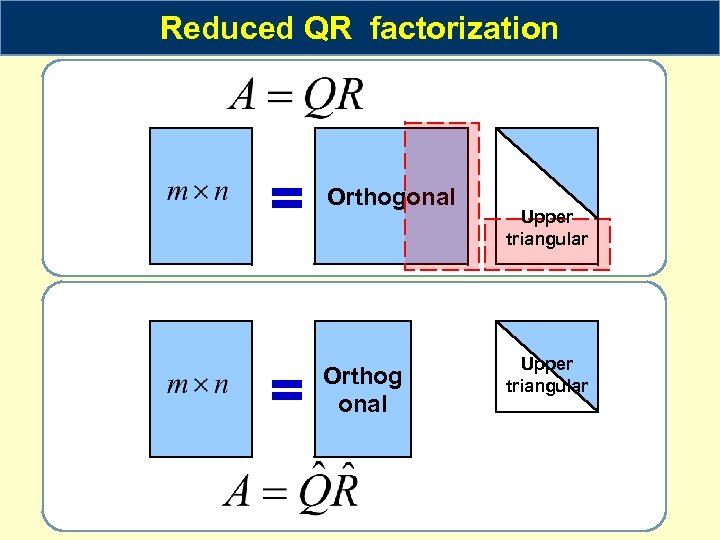Reduced QR factorization = Orthogonal = Orthog onal Upper triangular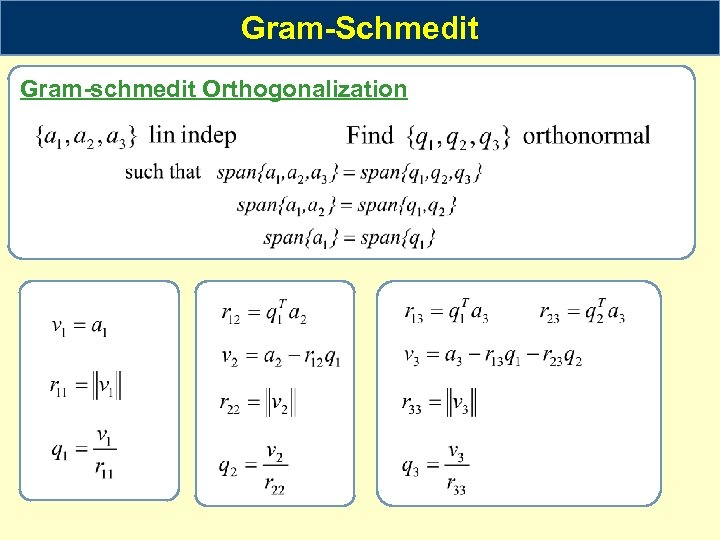Gram-Schmedit Gram-schmedit Orthogonalization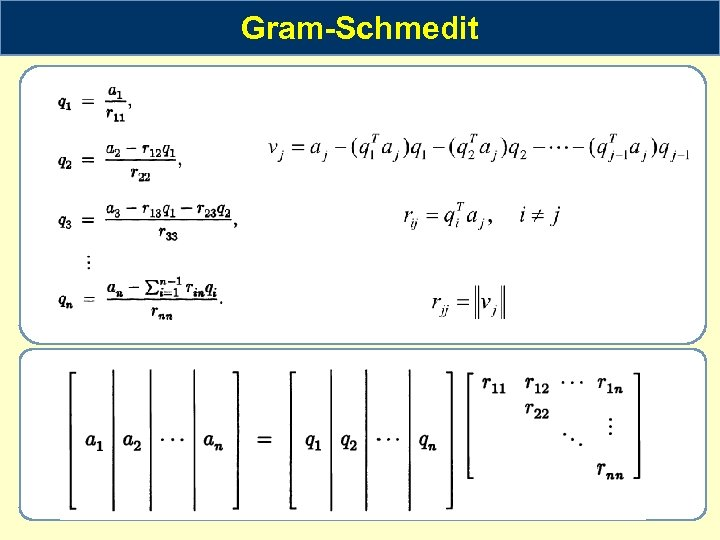Gram-Schmedit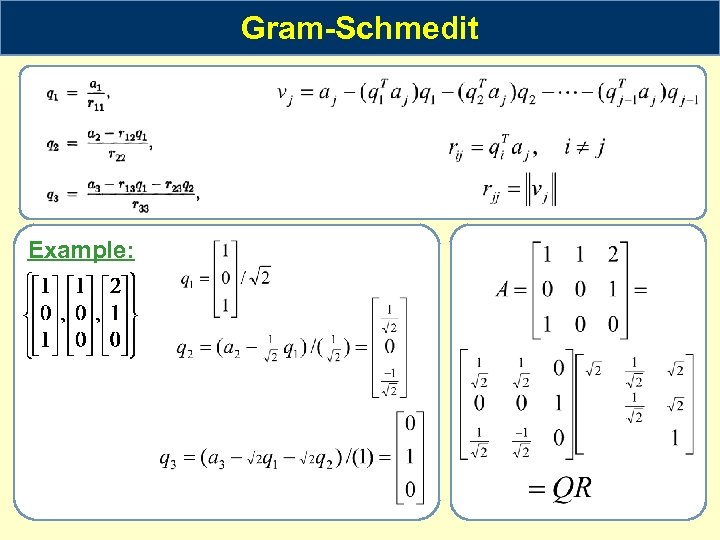Gram-Schmedit Example: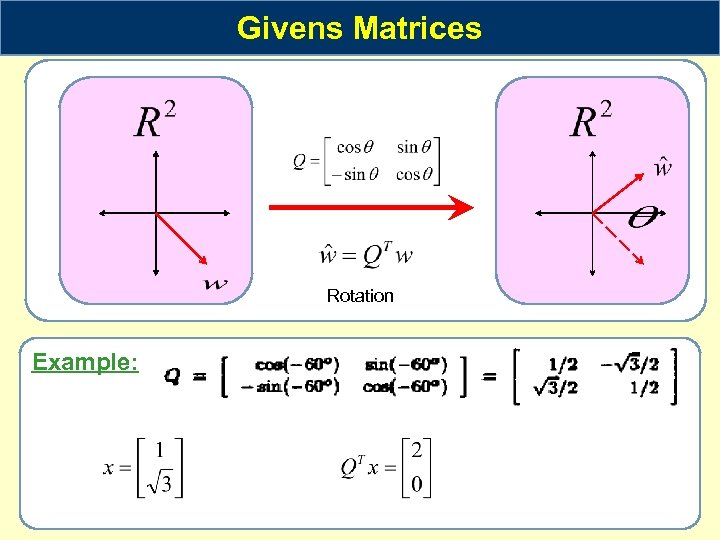Givens Matrices Rotation Example: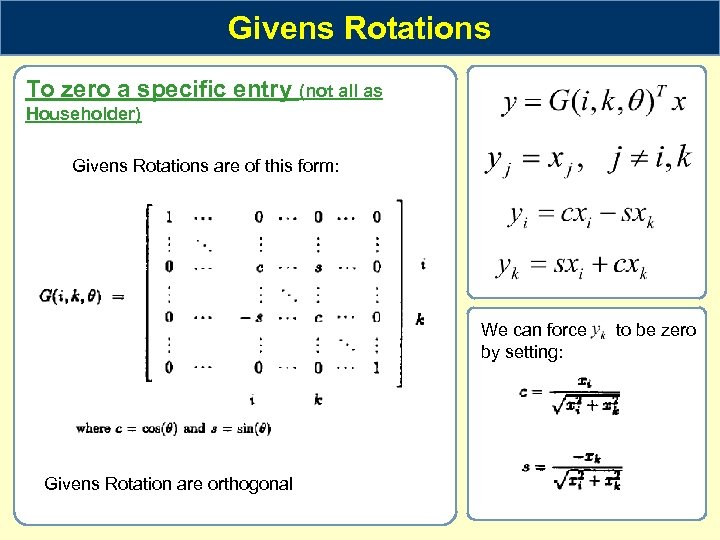Givens Rotations To zero a specific entry (not all as Householder) Givens Rotations are of this form: We can force by setting: Givens Rotation are orthogonal to be zero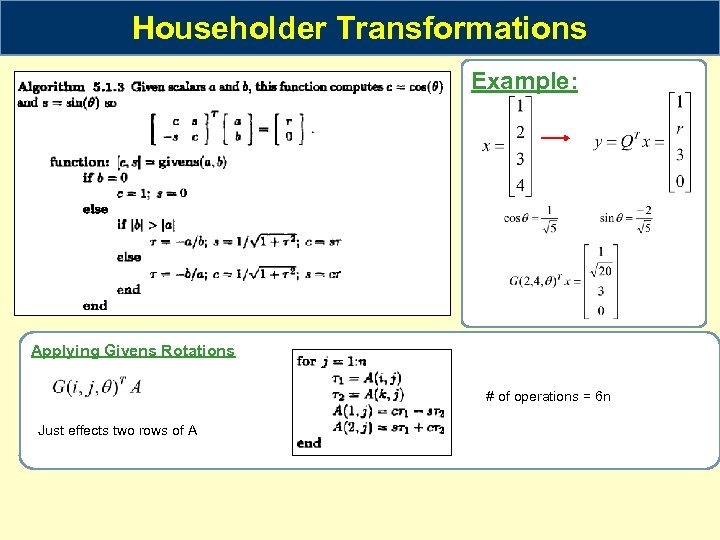Householder Transformations Example: Applying Givens Rotations # of operations = 6 n Just effects two rows of A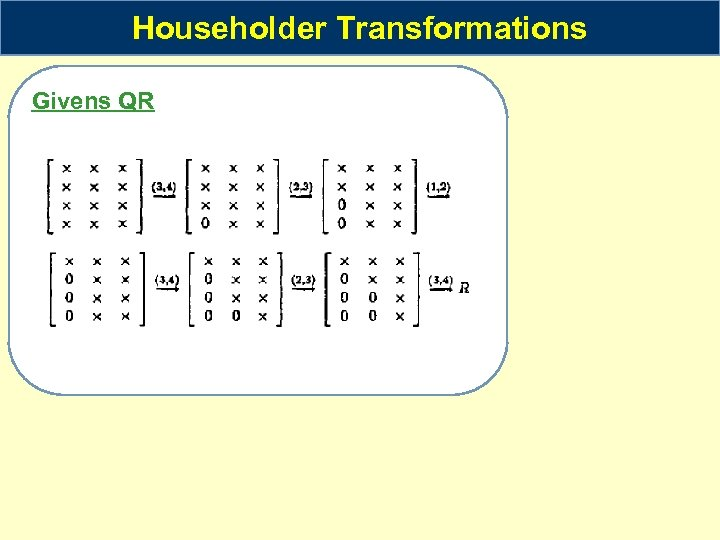Householder Transformations Givens QR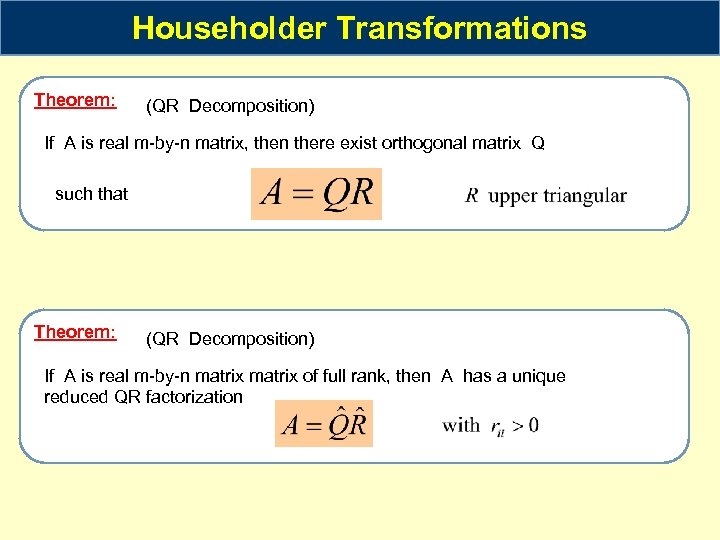Householder Transformations Theorem: (QR Decomposition) If A is real m-by-n matrix, then there exist orthogonal matrix Q such that Theorem: (QR Decomposition) If A is real m-by-n matrix of full rank, then A has a unique reduced QR factorization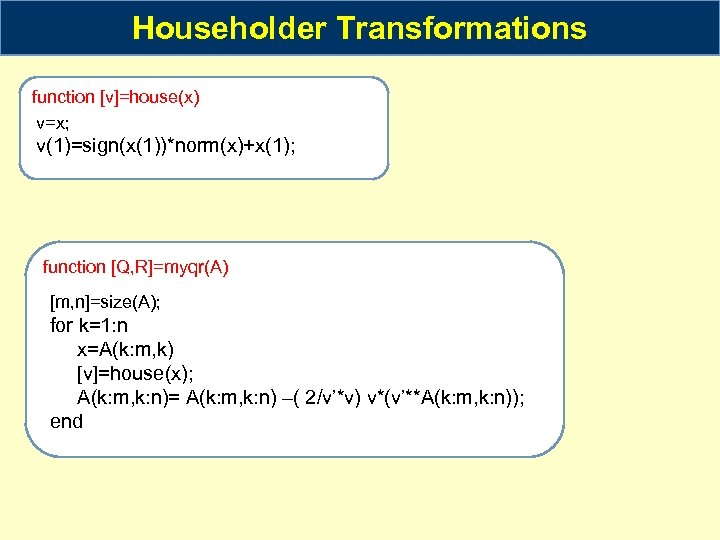Householder Transformations function [v]=house(x) v=x; v(1)=sign(x(1))*norm(x)+x(1); function [Q, R]=myqr(A) [m, n]=size(A); for k=1: n x=A(k: m, k) [v]=house(x); A(k: m, k: n)= A(k: m, k: n) –( 2/v’*v) v*(v’**A(k: m, k: n)); end